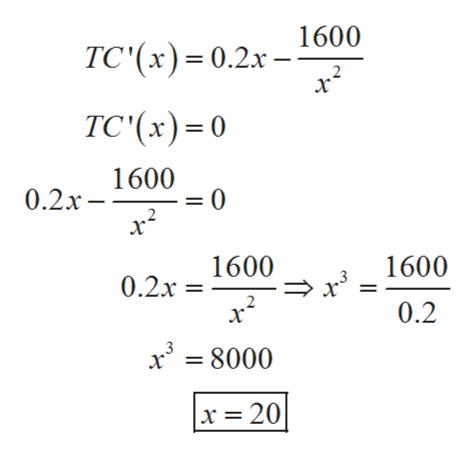# A company plans to manufacture a rectangular bin with a square base, an open top, and a volume of 800 cm3. The cost of the material for the base is 0.1 cents per square centimeter, and the cost of the material for the sides is 0.5 cents per square centimeter. Determine the dimensions of the bin that will minimize the cost of manufacturing it. What is the minimum cost?

Question
6 views

A company plans to manufacture a rectangular bin with a square base, an open top, and a volume of 800 cm3. The cost of the material for the base is 0.1 cents per square centimeter, and the cost of the material for the sides is 0.5 cents per square centimeter. Determine the dimensions of the bin that will minimize the cost of manufacturing it. What is the minimum cost?

check_circle

Step 1

According to the given information:

Let length of the rectangular bin be ‘x’ cm

Breadth of rectangular bin = ‘x’ cm

Height of rectangular bin = ‘y’ cm

Volume = 800 cm3

So,

Step 2

Cost of material for base = 0.1 cents per square centimeters

Cost of material for sides = 0.5 cents per square centimeters

So, the total cost for rectangular bin TC is:

Step 3

Now, to minimize the cost use sec...help_outlineImage Transcriptionclose1600 TC'(x0.2x TC'(x) 0 1600 = 0 0.2x- 1600 1600 0.2x 0.2 x= 8000 x = 20 fullscreen

### Want to see the full answer?

See Solution

#### Want to see this answer and more?

Solutions are written by subject experts who are available 24/7. Questions are typically answered within 1 hour.*

See Solution
*Response times may vary by subject and question.
Tagged in

### Derivative